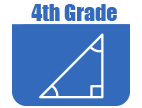IntroductionIn Grade 4, instructional time should focus on three critical areas: (1) developing understanding and fluency with multi-digit multiplication, and developing understanding of dividing to find quotients involving multi-digit dividends; (2) developing an understanding of fraction equivalence, addition and subtraction of fractions with like denominators, and multiplication of fractions by whole numbers; and (3) understanding that geometric figures can be analyzed and classified based on their properties, such as having parallel sides, perpendicular sides, particular angle measures, and symmetry.

(1) Students will generalize their understanding of place value to 1,000,000, understanding the relative sizes of numbers in each place. They will apply their understanding of models for multiplication (equal-sized groups, arrays, area models), place value, and properties of operations, in particular the distributive property, as they develop, discuss, and use efficient, accurate, and generalizable methods to compute products of multi-digit whole numbers. Depending on the numbers and the context, they will select and accurately apply appropriate methods to estimate or mentally calculate products. They will develop fluency with efficient procedures for multiplying whole numbers, understand and explain why the procedures work based on place value and properties of operations, and use them to solve problems. Students will apply their understanding of models for division, place value, properties of operations, and the relationship of division to multiplication as they develop, discuss, and use efficient, accurate, and generalizable procedures to find quotients involving multi-digit dividends. They will select and accurately apply appropriate methods to estimate and mentally calculate quotients, and interpret remainders based upon the context.

(2) Students will develop understanding of fraction equivalence and operations with fractions. They will recognize that two different fractions can be equal (for example, 15/9 = 5/3), and they will develop methods for generating and recognizing equivalent fractions. Students will extend previous understandings about how fractions are built from unit fractions, composing fractions from unit fractions, decomposing fractions into unit fractions, and using the meaning of fractions and the meaning of multiplication to multiply a fraction by a whole number.

(3) Students will describe, analyze, compare, and classify two-dimensional shapes. Through building, drawing, and analyzing two-dimensional shapes, students will deepen their understanding of properties of two-dimensional objects and the use of them to solve problems involving symmetry.The Online Core Resource pages are a collaborative project between the Utah State Board of Education and the Utah Education Network. If you would like to recommend a high quality resource, contact Trish French (Elementary) or Lindsey Henderson (Secondary). If you find inaccuracies or broken links contact resources@uen.org.